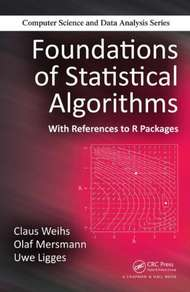## Foundations of Statistical AlgorithmsAuthors: Claus Weihs, Olaf Mersmann, Uwe Ligges Publisher: Chapman & Hall/CRC Published: December 27, 2013 Content: 474 Pages, 98 Illustrations Errata: fostal_errata.pdf

### Summary

A new and refreshingly different approach to presenting the foundations of statistical algorithms, Foundations of Statistical Algorithms: With References to R Packages reviews the historical development of basic algorithms to illuminate the evolution of today.s more powerful statistical algorithms. It emphasizes recurring themes in all statistical algorithms, including computation, assessment and verification, iteration, intuition, randomness, repetition and parallelization, and scalability. Unique in scope, the book reviews the upcoming challenge of scaling many of the established techniques to very large data sets and delves into systematic verification by demonstrating how to derive general classes of worst case inputs and emphasizing the importance of testing over a large number of different inputs.

Broadly accessible, the book offers examples, exercises, and selected solutions in each chapter as well as access to a supplementary website. After working through the material covered in the book, readers should not only understand current algorithms but also gain a deeper understanding of how algorithms are constructed, how to evaluate new algorithms, which recurring principles are used to tackle some of the tough problems statistical programmers face, and how to take an idea for a new method and turn it into something practically useful.

• Introduction
• Computation
• Motivation and History
• Models for Computing: What Can a Computer Compute?
• Floating-Point Computations: How Does a Computer Compute?
• Precision of Computations: How Exact Does a Computer Compute?
• Implementation in R
• Verification
• Motivation and History
• Theory
• Practice and Simulation
• Implementation in R
• Iteration
• Motivation
• Preliminaries
• Univariate Optimization
• Multivariate Optimization
• Example: Neural Nets
• Constrained Optimization
• Evolutionary Computing
• Implementation in R
• Deduction of Theoretical Properties
• PLS.from Algorithm to Optimality
• EM Algorithm
• Implementation in R
• Randomization
• Motivation and History
• Theory: Univariate Randomization
• Theory: Multivariate Randomization
• Practice and Simulation: Stochastic Modeling
• Implementation in R
• Repetition
• Motivation and Overview
• Model Selection
• Model Selection in Classification
• Model Selection in Continuous Models
• Implementation in R
• Scalability and Parallelization
• Introduction
• Motivation and History
• Optimization
• Parallel Computing
• Implementation in R
• Bibliography
• Index